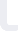Mathematica 9 for Beginners Udemy

# Mathematica 9 for Beginners

## Udemy

Price: USD 20

### Course details

This is an example based course aiming to teach Mathematica at an understandable level to students in college. Advanced high school students, or students whose high school teaches Mathematica will also find this course invaluable.

It assumes no understanding of programming languages, although knowledge, even rudimentary, of C/C++/Java is a plus.

This course does NOT teach Mathematica as a programming language.

This course does NOT teach Mathematics in general. Although a textbook on Pure Mathematics will come in handy for reference.

Any high school textbook that teaches college level Pure Mathematics is recommended. College students may use their course textbooks recommended by their Professor.

Students are expected to:

1. Know basic arithmetic and algebra including basic transcendental functions (exponential and trigonometric)

2. Know what a function is, including function composition, inverse and the definition of one-to-one functions

4. How to solve equations and inequalities.

3. Know common graphs of functions

4. Basic knowledge of set theory (preferably including partitioning and powersets)

5. Knowledge of matrices and vectors, preferably also knowledge of vector fields.

6. Rudimentary knowledge of 3D functions and functions on more than one variable

7. A broad knowledge of calculus including the basics of differential equations

8. Rudimentary knowledge of complex numbers

This course is in no way affiliated with Wolfram Research, Inc. The software, the Mathematica trademark and the associated logo belongs to Wolfram Research, Inc.

ScreenFlow, the software and associated trademarks belong to Telestream, Inc.

To contact me please e-mail: 2shakilrafi@gmail.com